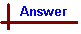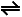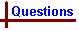.

 Problems involving Free Energy Your feedback on these self-help problems is appreciated. Click here to send an e-mail. Click here for a link to a data sheet Shortcut to QuestionsQ: 1 2 3 4 6 7 8 9 10 11 1 Calculation of ΔGo from ΔfHo and So tables. In each of the following, use the tables of ΔfHo and So to calculate ΔGo at 298 K for the reaction equation as given. (a) H2(g) + Cl2(g) → 2HCl(g) (b) C(graphite) + O2(g) → CO2(g) (c) N2(g) + O2(g) → 2NO(g) (d) N2(g) + 3H2(g) → 2NH3(g) (e) Cu(s) + ½O2(g) → CuO(s)2 Calculation of ΔGo from ΔfGo tables. Use ΔfGo tables to calculate ΔGo for each of the reactions in Question 1. Indicate in each case whether the reaction is spontaneous at 298 K and at standard conditions.3 Calculation of ΔG at non-standard conditions. Calculate ΔG at 298 K for the reaction 2NO(g) + O2(g) → 2NO2(g) for each of the following mixtures of the gaseous components. (a) P(NO(g)) = 0.50 atm, P(O2(g)) = 1.00 atm, P(NO2(g)) = 2.00 atm. (b) P(NO(g)) = 1.00 atm, P(O2(g)) = 2.00 atm, P(NO2(g)) = 0.50 atm. (c) P(NO(g)) = 0.10 atm, P(O2(g)) = 0.10 atm, P(NO2(g)) = 10.00 atm. (d) All pressures are 1.00 atm.4 For each of the mixtures in Q3 (a) - (d), is the reaction spontaneous towards the products side or the reactants side?5 Calculating the temperature at which spontaneous/non-spontaneous transition occurs At standard conditions and at 298 K, the reaction 2NO(g) + O2(g) → 2NO2(g) is spontaneous. Calculate the temperature at which it becomes non-spontaneous, assuming standard conditions apply.6 Calculation of K from ΔGo. Calculate K at 298 K for the reaction N2(g) + 3H2(g)2NH3(g) What does the value obtained indicate about the reaction and does this agree with your answer to Q2(d)?7 Calculate K at 298 K for the reaction N2(g) + O2(g)2NO(g) What does the value obtained indicate about the reaction and does this agree with your answer to Q2(c)?8 Additional problems A suggestion for solving the fuel requirement problem is to use electric power generated from solar energy to electrolyse water to form H2(g) and O2(g). The hydrogen then provides a pollution free fuel. (a) Calculate the enthalpy, entropy and thence the free energy change for the combustion of 2.00 g H2(g) to form H2O(l) at 298 K and 1 atm pressure. (b) Calculate the enthalpy, entropy and thence the free energy change for the combustion of 2.00 g of gaseous octane, C8H18(g), to form CO2(g) and H2O(l) at 298 K and 1 atm pressure. For C8H18(g), use fSo = 467 J K-1 mol-1 and ΔfHo = -208 kJ mol-1.9 (a) A bloke you met in a pub offers to sell you a catalyst that allows benzene to be formed by passing hydrogen gas over graphite at 25oC and one atmosphere pressure. Should you buy it? Why? (b) Is the reaction 2Fe(s) + 3/2O2(g) → Fe2O3(s) spontaneous at 298 K and one atmosphere pressure of oxygen gas? (c) Is the reaction to form glycine, 2CH4(g) + NH3(g) + 5/2O2(g) → H2NCH2COOH(s) + 3H2O(l) spontaneous at 298 K and one atmosphere pressure of the all gaseous components?10 Using standard enthalpy and entropy tables, determine the standard free energy change at 298 K for the reaction 2NO(g) + O2(g) → 2NO2(g)11 Use standard free energy of formation tables to calculate ΔGo for the reaction in the previous problem.12 A reaction is non-spontaneous at room temperature but is spontaneous at -40oC. What can you say about the signs and relative magnitude ofΔHo,ΔSo and TΔSo?13 Calculate ΔGo for the dissociation of HBrO, and thence ΔG for the dissociation when [H3O+] = 6.0 x 10-4 M, [BrO-] = 0.10 M and [HBrO] = 0.20 M. [Data: pKa(HBrO) = 8.64]14 (a) For the reaction at 298 K, N2(g) + 3H2(g) → 2NH3(g), using ΔfHo and ΔfGo tables, calculate: (i) ΔHo (ii) ΔGo (iii) K(298 K)   (b) For the reaction at 298 K H2(g) + I2(g) → 2HI(g) using ΔfHo and ΔfGo tables, calculate: (i) ΔGo (ii) ΔSo (iii) K   (c) The following gases are mixed, with the following partial pressures, at 298 K in a sealed container: H2(0.040 kPa), I2(0.040 kPa), and HI(0.080 kPa). For this non-standard system, calculate ΔG, and thence deduce the direction of spontaneous reaction for the system.15 From cell potentials, determine ΔGo for the reaction Zn(s) + Cl2(g, 1 at) → Zn2+(aq, 1M) + 2Cl-(aq, 1 M) Thence calculate K for the reaction.16 Using cell potentials, calculate ΔGo for the reaction Zn(s) + Cu2+(aq) → Zn2+(aq) + Cu(s)17 The following reactions can be coupled to produce alanine and oxalacetate. glutamate + pyruvateketoglutarate + alanine ΔGo'303 = -1.003 kJ mol-1. glutamate + oxalacetateketoglutarate + aspartate ΔGo'303 = -4.807 kJ mol-1. Write the form of the equilibrium constant for the following reaction and calculate the numerical value of the equilibrium constant at 303K. pyruvate + aspartatealanine + oxalacetateAnswers 1 (a) H2(g) + Cl2(g) → 2HCl(g) ΔHo = ΣΔfHo(products) - ΣΔfHo(reactants) = [2 x (-92.31)] - [0 + 0] = -184.6 kJ mol-1 ΔSo = ΣSo(products) - ΣSo(reactants) = [2 x (186.8)] - [(130.6) + (223.0)] = 20.0 J K-1 mol-1 or 0.0200 kJ K-1 mol-1 [Note the difference in the units of S and H] ΔGo = ΔHo - TΔSo = -184.6 - 298 x 0.0200 = -191 kJ mol-1     (b) C(graphite) + O2(g) → CO2(g) ΔHo = ΣΔfHo(products) - ΣΔfHo(reactants) = [(-393.5)] - [0 + 0] = -393.5 kJ mol-1 ΔSo = ΣSo(products) -ΣSo(reactants) = [(213.7)] - [(5.686) + (205.0)] = 3.014 J K-1 mol-1 or 0.003014 kJ K-1 mol-1 ΔGo = ΔHo - TΔSo = -393.5 - 298 x 0.003014 = -393 kJ mol-1   (c) N2(g) + O2(g) → 2NO(g) ΔHo = ΣΔfHo(products) -ΣΔfHo(reactants) = [2 x (90.29)] - [0 + 0] = 180.6 kJ mol-1 ΔSo = ΣSo(products) -ΣSo(reactants) = [2 x (210.65)] - [(191.5) + (205.0)] = 24.9 J K-1 mol-1 or 0.0249 kJ K-1 mol-1 ΔGo = ΔHo - TΔSo = 180.6 - 298 x 0.0249 = 173 kJ mol-1   (d) N2(g) + 3H2(g) → 2NH3(g) ΔHo = ΣΔfHo(products) -ΣΔfHo(reactants) = [2 x (-45.9)] - [0 + 0] = -91.8 kJ mol-1 ΔSo = ΣSo(products) -ΣSo(reactants) = [2 x (193)] - [(191.5) + 3 x (130.6)] = -197.3 J K-1 mol-1 or -0.1973 kJ K-1 mol-1 ΔGo = ΔHo - TΔSo = -91.8 - 298 x (-0.1973) = -33 kJ mol-1   (e) Cu(s) + ½O2(g) → CuO(s) ΔHo = ΣΔfHo(products) -ΣΔfHo(reactants) = [(-157.3)] - [0 + 0] = -157.3 kJ mol-1 ΔSo = ΣSo(products) -ΣSo(reactants) = [(42.63)] - [(33.1) + ½ x (205.0)] = -93.0 J K-1 mol-1 or -0.0930 kJ K-1 mol-1 ΔGo = ΔHo - TΔSo = -157.3 - 298 x (-0.0930) = -130 kJ mol-12 (a) H2(g) + Cl2(g) → 2HCl(g) ΔGo = ΣΔfGo(products) -ΣΔfGo(reactants) = [2 x (-95.3)] - [0 + 0] = -191 kJ mol-1 As the sign is negative, this reaction is spontaneous at 298 K and standard conditions.   (b) C(graphite) + O2(g) → CO2(g) ΔGo = ΣΔfGo(products) -ΣΔfGo(reactants) = [(-394.4)] - [0 + 0] = -394 kJ mol-1. As the sign is negative, this reaction is spontaneous at 298 K and standard conditions.   (c) N2(g) + O2(g) → 2NO(g) ΔGo = ΣΔfGo(products) -ΣΔfGo(reactants) = [2 x (86.6)] - [0 + 0] = 173 kJ mol-1 As the sign is positive, this reaction is non-spontaneous at 298 K and standard conditions.   (d) N2(g) + 3H2(g) → 2NH3(g) ΔGo = ΣΔfGo(products) -ΣΔfGo(reactants) = [2 x (-16.0)] - [0 + 0] = -32 kJ mol-1 As the sign is negative, this reaction is spontaneous at 298 K and standard conditions.   (e) Cu(s) + ½O2(g) → CuO(s) ΔGo = ΣΔfGo(products) -ΣΔfGo(reactants) = [(-130)] - [0 + 0] = -130 kJ mol-1 As the sign is negative, this reaction is spontaneous at 298 K and standard conditions.3 2NO(g) + O2(g) → 2NO2(g) ΔGo = ΣΔfGo(products) -ΣΔfGo(reactants) = [2 x (51)] - [2 x (86.6) + 0] = -71.2 kJ mol-1 ΔG = ΔGo + RTlnQ = -71.2 + ((8.314 x 298 x lnQ))/1000 kJ mol-1 [Note: The value of R is 8.314 J K-1 mol-1 and is divided by 1000 to match the units of J for G] = -71.2 + 2.4776 x lnQ kJ mol-1.   (a) Q = P(NO2)2 / (P(NO)2 x P(O2)) = (2.00)2 /((0.50)2 x (1.00)) = 4/0.25 = 16 ΔG = -71.2 + 2.4776 x ln(16) = -64 kJ mol-1   (b) Q = P(NO2)2/(P(NO)2 x P(O2)) = (0.50)2 /((1.00)2 x (2.00)) = 0.25/2.00 = 0.125 ΔG = -71.2 + 2.4776 x ln(0.125) = -76 kJ mol-1   (c) Q = P(NO2)2/((P(NO)2 x P(O2)) = (10.00)2/((0.10)2 x (0.10)) = 100/(1.0 x 10-3) = 1.0 x 105 ΔG = -71.2 + 2.4776 x ln(1.0 x 105) = -43 kJ mol-1     (d) Q = P(NO2)2/(P(NO)2 x P(O2)) = (1.00)2/((1.00)2 x (1.00)) = 1.00 / 1.00 = 1.00 ΔG = -71.2 + 2.4776 x ln(1.00) = -71.2 kJ mol-1 [Alternatively, given that all pressures are at the standard state of 1 atm, ΔG = ΔGo = -71.2 kJ mol-1]4 In all cases, the sign of ΔG is negative, so the reaction would proceed to the right for each of the mixtures used.5 2NO(g) + O2(g) → 2NO2(g) ΔGo = ΔHo - TΔSo ΔHo = [2 x 33.2] - [2 x (90.3) + 0] = -114.2 kJ mol-1 ΔSo = [2 x (239.9)] - [2 x (210.65) + 205] = -146.5 J K-1 mol-1 [Note the units of H are kJ mol-1 while those of S are J K-1 mol-1] The change spontaneous to/from non-spontaneous occurs at the temperature such that ΔGo = 0 i.e. when ΔHo = TΔSo or at T = ΔHo/ΔSo = -114.2 x 103/-146.5 = 780 K6 N2(g) + 3H2(g)2NH3(g) At equilibrium, ΔG = 0, ΔGo = -RTlnK From Q2, ΔGo = -32 kJ mol-1 or -32 ´ 103 J mol-1 -32 x 103 = -8.314 x 298 x lnK lnK = 12.916 K = 4.1 x 105 The large value of K indicates that the equilibrium lies to the right as indicated by the value of ΔGo from Q2.7 N2(g) + O2(g)2NO(g) At equilibrium, ΔG = 0, ΔGo = -RTlnK From Q2, ΔGo = +173 kJ mol-1 173 x 103 = -8.314 x 298 x lnK lnK = -69.826 K = 4.7 x 10-31 The extremely small value of K indicates that the equilibrium lies to the left as indicated by the value of ΔGo from Q2.8 (a) The reaction equation is H2(g) + ½O2(g) → H2O(l) 2.00 g hydrogen = 1.00 mole, so calculate ΔHo and ΔSo for the equation as written. ΔHo = [-286] -  = -286 k J mol-1 ΔSo =  - [131 + ½ x 205] = -163.5 J K-1 mol-1 ΔGo = ΔHo - TΔSo = -286 x 103 -298 x (-163.5) = -237 x 103 J mol-1 or -237 kJ mol-1   (b) Calculate the quantities for the equation as written with one mole of octane and then multiply by the fraction of a mole that 2.00 g of octane represents. C8H18(g) + 12½O2(g) → 8CO2(g) + 9H2O(l) ΔHo = [8 x (-394) + 9 x (-286)] - [(-208) + 0] = -5518 kJ mol-1 GFW of octane = 114.2 g mol-1 2.00 g = 2.00/114.2 mole = 0.0175 mole Enthalpy change for 2.00 g octane = 0.0175 x -5518 = -96.7 kJ ΔSo = [8 x (214) + 9 x (70)] - [(467) + 12½ x (205)] = 2342 - 3029.5 = -687.5 J K-1 mol-1 For 2.00 g octane, entropy change = 0.0175 x -687.5 = -12.0 J K-1 ΔGo = ΔHo - TΔSo = -96.7 x 103 - 298 x (-12.0) = -93.1 x 103 J or -93.1 kJ. The free energy change is much greater for hydrogen on a weight basis.9 (a) For the reaction 6C(s) + 3H2(g) → C6H6(l) at 298 K ΔGo = [(130)] - [0 + 0] = +130 kJ mol-1 The + sign shows this is not a spontaneous reaction at these conditions, therefore do not buy.   (b) For the reaction Fe(s) + 1½O2(g) → Fe2O3(s) at 298 K ΔGo = [(-742] - [0 + 0] = -742 kJ mol-1 The - sign shows this is a spontaneous reaction at these conditions.   (c) For the reaction at 298 K 2CH4(g) + NH3(g) + 2½O2(g) → NH2CH2COOH(s) + 3H2O(l) ΔGo = [(-369 + 3 x (-237)] - [2 x (-51) + (-16) + 0] = -962 kJ mol-1 The - sign shows this is a spontaneous reaction at these conditions.10 The reaction equation is 2NO(g) + O2(g) → 2NO2(g) ΔHo = [2 x (33.2)] - [2 x (90.3) + 0] = -114.2 k J mol-1 ΔSo = [2 x (239.9)] - [2 x (210.7) + (205)] = -146.6 J K-1 mol-1 ΔGo = ΔHo - TΔSo = -114.2 x 103 - 298 x (-146.6) = -71 x 103 J mol-1 or -71 kJ mol-111 Using ΔGo tables in the Data section, ΔGo = [2 x (51.0)] - [2 x (86.6)] = -71 kJ mol-112 ΔGo becomes negative at T < 233 K but is +ve above this temperature. ΔHo is regarded as not changing significantly with temperature, so in order for ΔHo - TΔSo to be positive at T > 233 K but -ve at T < 233 K, ΔHo must be -ve and ΔSo also must be -ve. Then at T > 233 K, the magnitude of TΔSo exceeds that of ΔHo, giving a +ve value for ΔGo. At T < 233 K, the -ve ΔHo exceeds the TΔSO, leaving an overall -ve value for ΔGo. (Goet iT?)13 The reaction equation is HBrO + H2OH3O+ + BrO- for which K = Ka = 10-8.64 ΔGo = -RTlnK = -8.314 x 298 x ln(10-8.64) = -8.314 x 298 x (-19.89) = 4.93 x 104 J mol-1 or 49.3 kJ mol-1 ΔG = ΔGo + RTlnQ If [H3O+] = 6.0 x 10-4 M, [BrO-] = 0.10 M and [HBrO] = 0.20 M, then ΔG = 49.3 x 103 (J) + 8.314 x 298 x ln((6.0 x 10-4)(0.10)/ (0.20)) [Note the use of R = 8.314 J K-1 mol-1 to match the units of ΔG and the conversion of the units of ΔG to J to match the RTlnK term] = 49.3 x 103 + 8.314 x 298 x ln(3.0 x 10-4) J mol-1 = 49.3 x 103 + 8.314 x 298 x (-8.111) J mol-1 = 49.3 x 103 + (-20.1 x 103) J mol-1 = 29.2 x 103 J mol-1 or 29.2 kJ mol-114 (a) (i) N2(g) + 3H2(g) → 2NH3(g) ΔHo = ΣΔfHo(products) -ΣΔfHo(reactants) = [2 x (-45.9)] - [0 + 0] = -91.8 kJ mol-1 (ii)ΔGo = ΣΔfGo(products) - ΣD fGo(reactants) = [2 ´ (-16.5)] - [0 + 0] = -33.0 kJ mol-1 (iii)ΔGo = -RTlnK From (ii), ΔGo = -33.0 kJ mol-1 -33.0 x 103 (J) = -8.314 x 298 x lnK lnK = 13.314 K = 6.09 x 105 (b) (i) H2(g) + I2(g) ® 2HI(g) ΔGo = ΣΔfGo(products) -ΣΔfGo(reactants) = [2 x (1.7)] - [0 + -19.4] = -16.0 kJ mol-1 To calculate ΔSo, it is first necessary to calculate ΔHo ΔHo = ΣΔfHo(products) -ΣΔfHo(reactants) = [2 x (27.0)] - [0 + 62.0] = -8.0 kJ mol-1 Then ΔGo = ΔHo - TΔSo provides -16.0 = -8.0 - 298 ´ ΔSo ΔSo = -8.0/298 = 2.68 ´ 10-2 kJ K-1 mol-1 or 26.8 J K-1 mol-1 (c) H2(g) + I2(g) → 2HI(g) From (b)(i) above, ΔGo = -16.0 kJ mol-1 At non-standard conditions, ΔG = ΔGo + RTlnQ Q = P(HI)2 / (P(H2) x P(I2)) = (0.080)2 /((0.040) x ( 0.040)) = 4.0 ΔG = -16.0 + (8.314 ´ 298 ´ ln(4.0))/1000 kJ mol-1 [Note: Must use R = 8.314 to match the units of J in ΔG and must divide the RTlnQ term by 1000 to convert it to kJ to match the units of ΔG] = -12.6 kJ mol-1 As ΔG is negative, the reaction is spontaneous from left to right as written in the equation above.15 The overall reaction equation is Zn(s) + Cl2(g) → Zn2+(aq,1 M) + 2Cl-(aq, 1 M) From the half reactions Zn2+ + 2e-Zn Eo = -0.76 V Cl2 + 2e-2Cl- Eo = 1.36 V Eocell = 1.36 - (-0.76) V = 2.12 V ΔGo = -nFEocell = -2 x 96500 x 2.12 J mol-1 = -409 x 103 J mol-1 or -409 kJ mol-1 ΔGo = -RTlnK -409 x 103 = -8.314 x 298 x lnK lnK = 165.08 K = 4.9 x 107116 The overall reaction equation is Zn(s) + Cu2+(aq) → Zn2+(aq) + Cu(s) From the half reactions Zn2+ + 2e-Zn Eo = -0.76 V Cu2+ + 2e-Cu Eo = 0.34 V Eocell = 0.34 - (-0.76) V = 1.10 V ΔGo = -nFEocell = -2 x 96500 x 1.10 J mol-1 = -212 x 103 J mol-1 or -212 kJ mol-117 Add the reverse of the second equation to the first equation. pyruvate + aspartatealanine + oxalacetate ΔGo'303 = (-1.003) + (+4.807) = 3.804 kJ mol-1 ΔGo'303 = -RTlnK 3.804 x 103 = -8.314 x 303 x lnK lnK = -3.804 x 103 /(8.314 x 303) = -1.51 K = 0.22